2023-08-09 14:53:19

# 语法分析

## 一、ANTLR 4 语法分析器

### 1. 二义性文法

• 二义性是不能接受的，必须消除

#### 1.1 IfStat

Q: 如何判断文法有没有二义性

A: 做不到, 只能靠经验

Q: ANTLR 如何解决二义性问题

A: 通过书写规则的顺序来决定优先级, 优先解释为优先级高的结构

#### 1.2 Left-Factoring

• 很明显, 提取左公因子无助于消除文法二义性
• ANTLR 4 可以处理有左公因子的文法

#### 1.3 Expr

• 左递归(左结合)，右递归(右结合)
• ANTLR 4 可以处理(直接)左递归
• 写在上方的规则优先级高
• 但是有些情况需要右结合(^)
• 二元运算符需要特别标记 <assoc = right> expr '^' expr
• 一元运算符不需要标记，因为只能解释为右结合 ------1

### 2. Call Graphs

#### 2.1 监听器模式(Listener)

• ANTLR 通过深度优先遍历生成的语法树

• 除了叶子节点, 所有的内部节点至少会经过两次
• ANTLR 会为其生成 ENTERXXX(), EXITXXX() 的方法
• 一条规则有不同选择时, 通过添加 #label 来区分, 单个选择内部使用 op 来区分

• ANTLR 会生成对应的 ENTER\EXIT LABEL 方法, op 则作为成员变量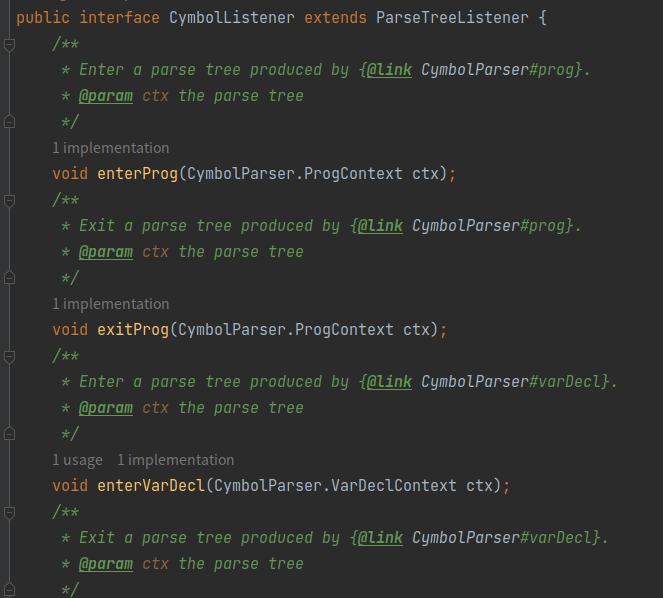#### 2.2 访问者模式(Visitor)

• 详见实验

• Visitor在访问每个节点的子节点前会调用visitChildren()函数

• Visitor在访问每个终结符节点时会调用visitTerminal()函数

• 对于每一个语法规则都存在一个对应的visit函数，如 exp 规则对应的函数为visitExp()

### 3. 上下文无关文法

#### 3.1 语法

##### Definition (Context-Free Grammar (CFG); 上下文无关文法)

• $T$ 是终结符号 (Terminal) 集合, 对应于词法分析器产生的词法单元;

• $N$ 是非终结符号 (Non-terminal) 集合;

• $P$ 是产生式 (Production) 集合;

• $A \in N \longrightarrow α \in (T \cup N)^\ast$

• 头部/左部 (Head) $A$: 单个非终结符

• 体部/右部 (Body) $α$: 终结符与非终结符构成的串, 也可以是空串 $\epsilon$

• $S$ 为开始 (Start) 符号。要求 $S \in N$ 且唯一。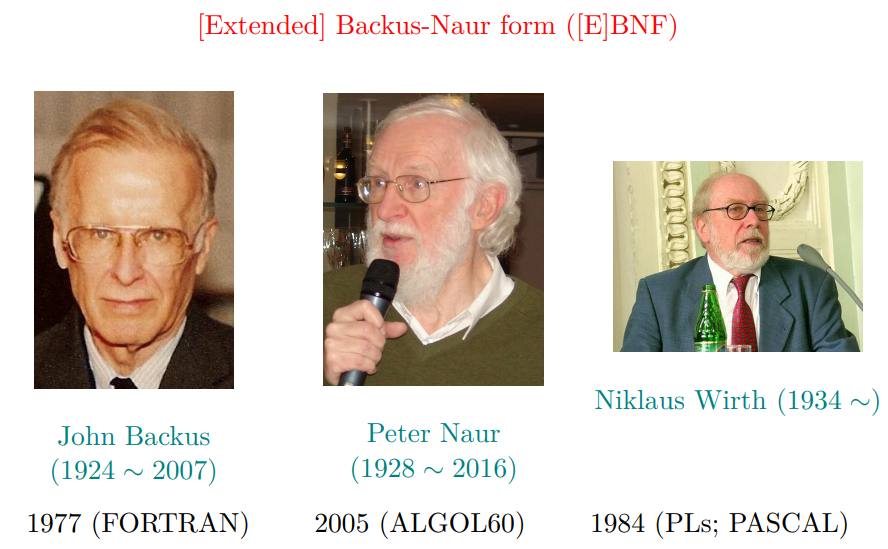#### 3.2 语义

• 语言是的集合
• 串从何来?
##### 推导 (Derivation)
• 推导即是将某个产生式的左边替换成它的右边
• 每一步推导需要选择替换哪个非终结符号, 以及使用哪个产生式

$E \to E + E \mid E \ast E \mid (E) \mid -E \mid \bf id$

• $E \implies -E \implies -(E) \implies -(E + E) \implies -(\textbf{id} + E) \implies -(\textbf{id} + \textbf{id})$
• $E \implies -E \implies -(E) \implies -(E + E) \implies \textcolor{blue}{-(E + \textbf{id})} \implies -(\textbf{id} + \textbf{id})$

$E \implies -E:$ 经过一步推导得出

$E \stackrel{+}{\implies} -(\textbf{id} + E):$ 经过一步或多步推导得出

$E \stackrel{\ast}{\implies} -(\textbf{id} + E):$ 经过零步或多步推导得出

##### Definition (Sentential Form; 句型)

• $E \to E + E \mid E \ast E \mid (E) \mid -E \mid \bf id$
• $E \implies -E \implies -(E) \implies -(E + E) \implies -(\textbf{id} + E) \implies -(\textbf{id} + \textbf{id})$
• 夹杂着终结符和非终结符的串都叫做句型

##### Definition (文法 $G$ 生成的语言 $L(G)$)

• $w \in L(G) \iff S \stackrel{\ast}{\Rightarrow} w$

#### 3.3 关于文法 $G$ 的两个基本问题

• Membership问题: 给定字符串 $x \in \textcolor{red}{T^\ast} , x \in L(G)$ ?

• $L(G)$ 究竟是什么?

##### 3.3.1 给定字符串 $x \in T^\ast , x \in L(G)$ ?
• 即, 检查 $x$ 是否符合文法 $G$

• 这就是语法分析器的任务:

• 为输入的词法单元流寻找推导、构建语法分析树, 或者报错
##### 3.3.2 $L(G)$ 是什么?
• 这是程序设计语言设计者需要考虑的问题

• 一些例子

• $L(G) = \set{良匹配括号串}$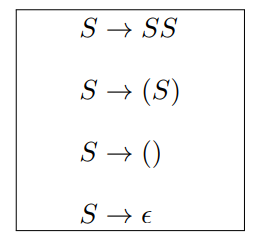• $L(G) = \set{a^nb^n \mid n \ge 0}$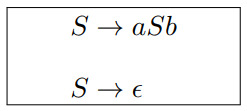• $字母表 \Sigma = \set{a, b} 上的所有 \textcolor{blue}{\textbf{回文串}} (Palindrome) 构成的语言$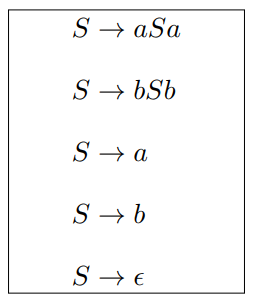• $\set{b^na^mb^{2n} \mid n \ge 0, m \ge 0}$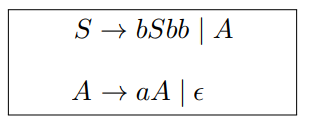• $\set{x \in \set{a, b}^\ast \mid x 中 a, b 个数 \textcolor{red}{\textbf{相同}}}$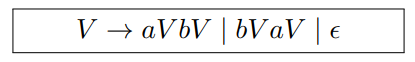• $a, b$ 开头是对称的, 此处只考虑 $a$ 开头
• 一定存在一个 $b$ 使得按这个 $b$ 分割的两个串中 $a, b$ 个数相同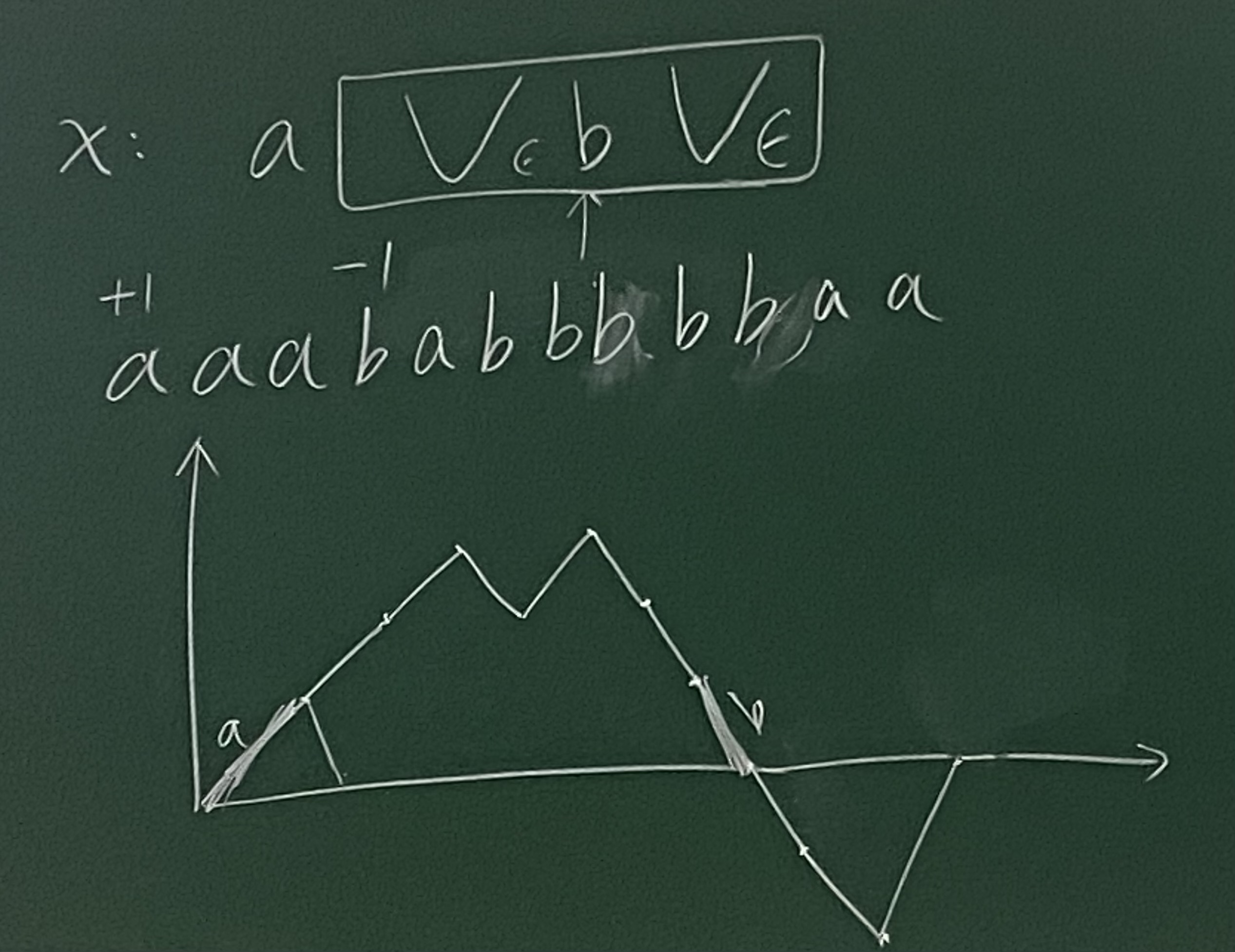• $\set{x \in \set{a, b}^\ast \mid x 中 a, b 个数 \textcolor{red}{\textbf{不同}}}$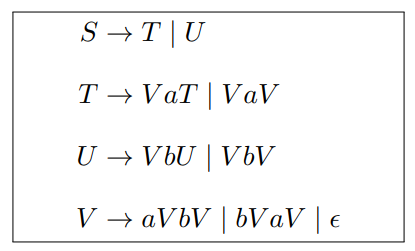### 4 正则表达式

• 正则表达式的表达能力严格弱于上下文无关文法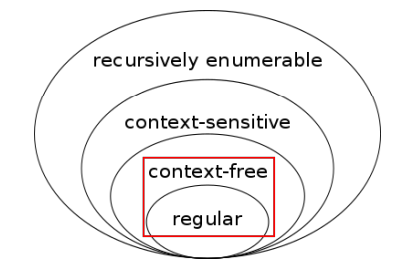#### 4.1 RE $\rightarrow$ CFG

• RE

• $r = (a \mid b)^\ast abb$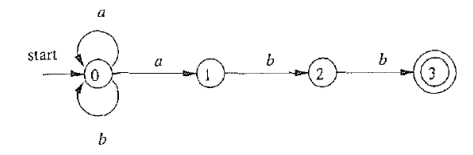• CFG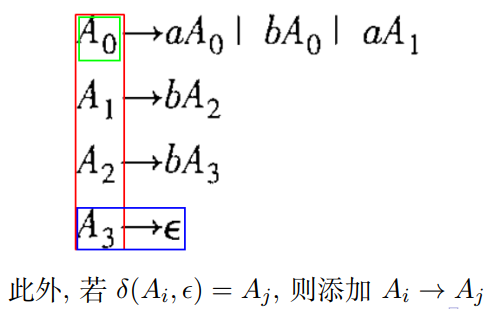#### 4.2 CFG $\rightarrow$ RE 反例

• CFG

• $L = \set{a^nb^n \mid n \ge 0}$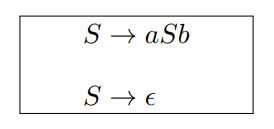• RE

• 该语言无法使用正则表达式来描述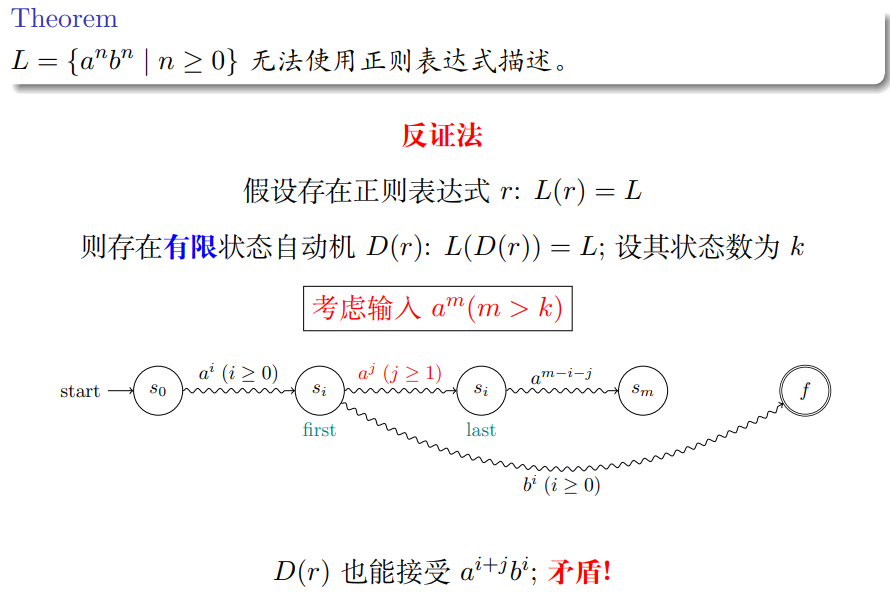• 因为 $m \gt k$, 所以一定会有状态经过了不止一次, 假设就是 $s_i$, 上图画出第一次碰到和最后一次碰到的状态

• 第一次到最后一次中间消耗的 $a$ 的个数一定 $\ge 1$
• 假设消耗掉 $a^i$ 后第一次到达 $s_i$, 此时接收 $b^i$ 后根据假设应当到达终止状态 $f$

• 但因为第一次到达和最后一次到达的 $s_i$ 是相同的状态, 因此也应当可以接受 $a^{i+j}b^i$
• 矛盾！
• 该反例称为泵引理, Pumping Lemma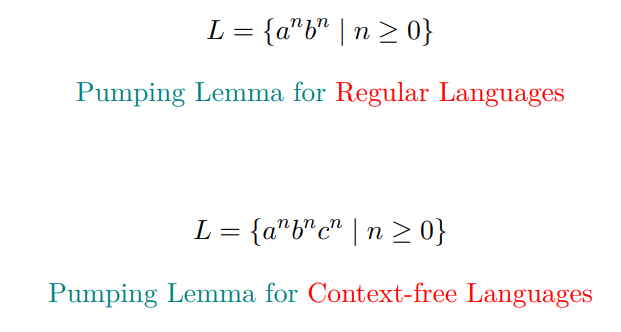## 二、递归下降的$LL(1)$语法分析器

### 1. 背景介绍

• $LR$ 更加复杂

• $LL$ 为自顶向下
• $LR$ 为自底向上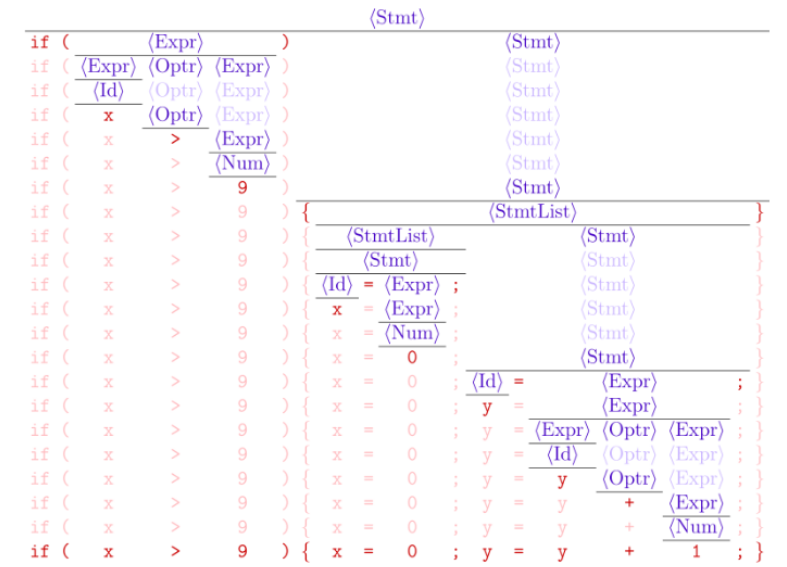• 这意味着, 每个句子对应唯一的一棵语法分析树
• 本节主题: $LL(1)$ 语法分析器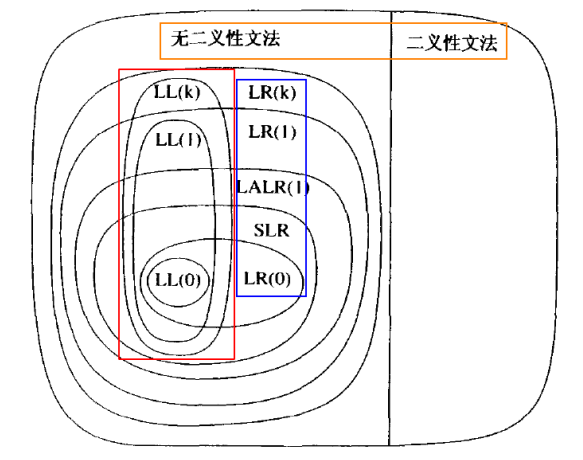ANTLR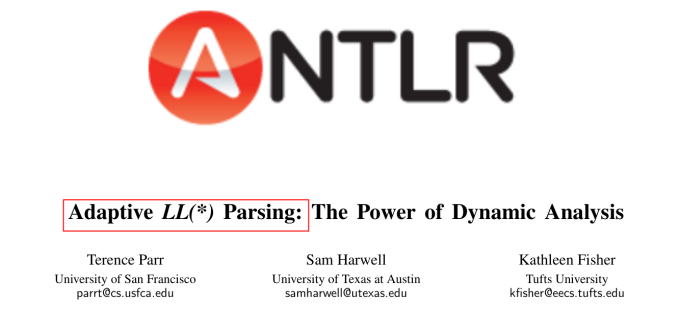### 2. 什么是$LL(1)$语法分析器

• 自顶向下的、
• 递归下降的、
• 基于预测分析表的、
• 适用于$\textcolor{red}{LL(1) \textbf{文法}}$的、
• $LL(1)$ 语法分析器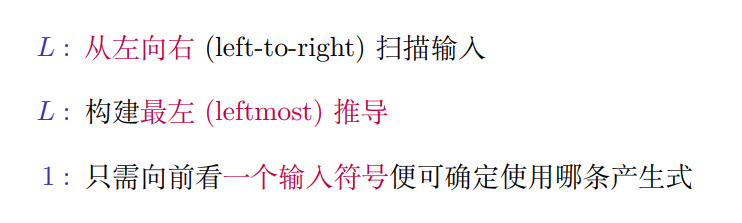#### 2.1 自顶向下

$\textcolor{red}{\textbf{自顶向下}}构建语法分析树$

• $\textcolor{blue}{\textbf{根节点}}是文法的起始符号$ $S$
• $每个\textcolor{blue}{\textbf{中间节点}}表示\textcolor{purple}{\textbf{对某个非终结符应用某个产生式进行推导}}$
• $\textcolor{red}{Q:}选择哪个非终结符, 以及选择哪个产生式$
• $\textcolor{blue}{\textbf{叶节点}}是词法单元流$ $w$$•$仅包含终结符号与特殊的\textcolor{teal}{\textbf{文件结束符}}\textcolor{teal}{$}$ $\textcolor{teal}{\texttt{(EOF)}}$
##### 如何选择非终结符
• 在推导的每一步, $L\textcolor{red}{L}(1)$ 总是选择最左边的非终结符进行展开
• Leftmost derivation, 最左推导
• $\textcolor{red}{L}L(1)$: 从左向右读入词法单元

#### 2.2 递归下降

$\textcolor{red}{\textbf{递归}}\textcolor{blue}{\textbf{下降}}的典型实现框架$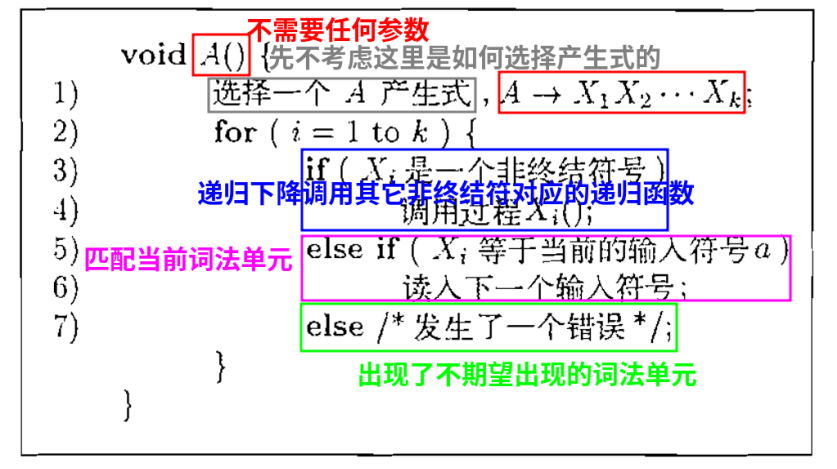• 为每个非终结符写一个递归函数
• 内部按需调用其它非终结符对应的递归函数, 下降一层
##### 递归下降示例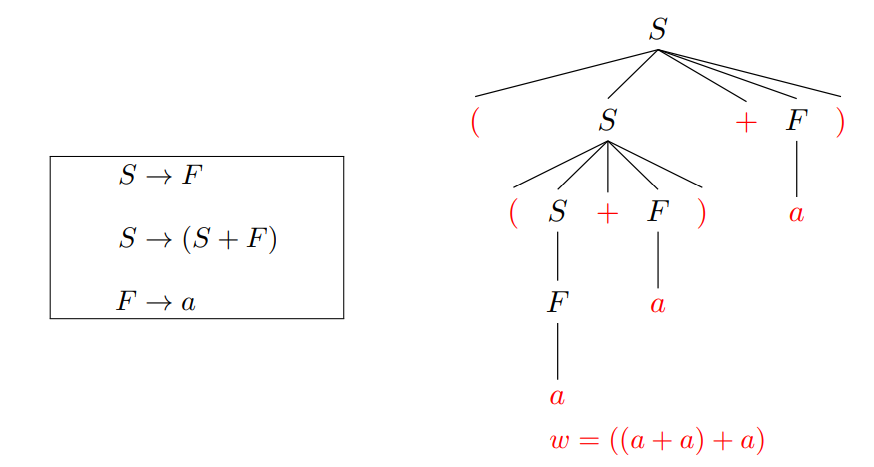• 每次都选择语法分析树最左边的非终结符进行展开

$Q: 同样是展开非终结符 S, 为什么前两次选择了 S \to (S + F), 而第三次选择了 S \to F$ $?$

• 因为它们面对的当前词法单元不同

#### 2.3 预测分析表

$使用\textcolor{red}{\textbf{预测分析表}}确定产生式$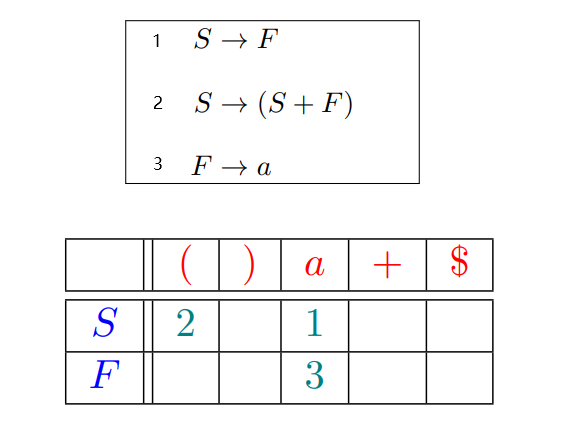• $指明了每个\textcolor{blue}{\textbf{非终结符}}在面对不同的\textcolor{red}{\textbf{词法单元或文件结束符}}时,$

$该选择哪个\textcolor{teal}{\textbf{产生式}} (按编号进行索引) 或者\textcolor{cyan}{\textbf{报错}} (空单元格)$

#### 2.4 Definition ($LL(1)$ 文法)

$如果文法$ $G$ 的$\textcolor{red}{\textbf{预测分析表}}是\textcolor{blue}{\textbf{无冲突}}的, 则$ $G$ $是$ $LL(1)$ $文法$

• $\textcolor{blue}{\textbf{无冲突}}: 每个单元格里只有一个产生式 (编号)$

• $对于当前选择的\textcolor{blue}{\textbf{非终结符}},$

$仅根据输入中\textcolor{red}{\textbf{当前的词法单元}} (LL(\textcolor{red}{1}))$ $即可确定需要使用哪条\textcolor{teal}{\textbf{产生式}}$

##### 递归下降的、预测分析实现方法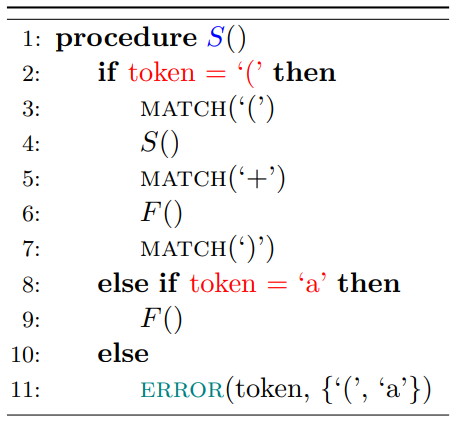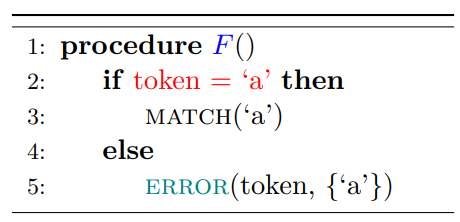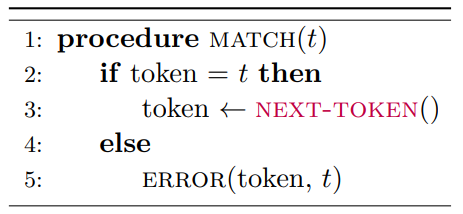### 3. 计算给定文法 $G$ 的预测分析表

#### 3.1 引入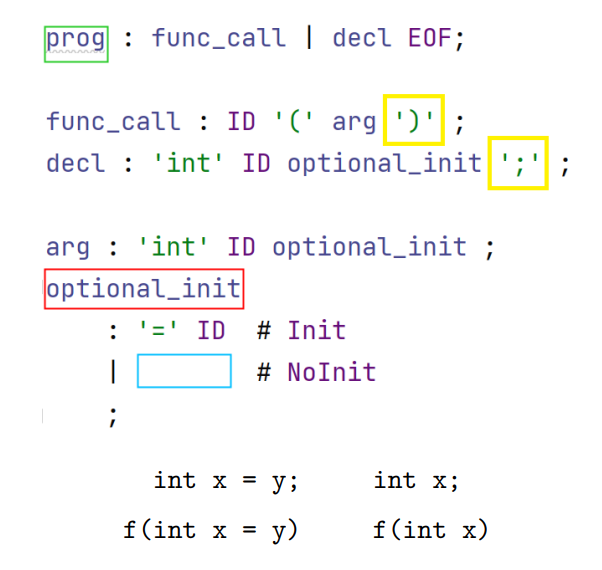• 重点在于 optional_init 展开时如何选择
• 非终结符展开要考虑两件事
• 最左侧的终结符是不是所需要的
• 如果要展开的非终结符的一个备选分支是 $\epsilon$, 后面的字符是不是所需要的

#### 3.2 FIRST($\alpha$)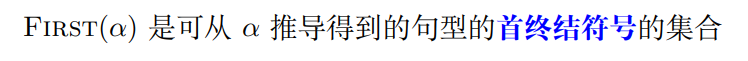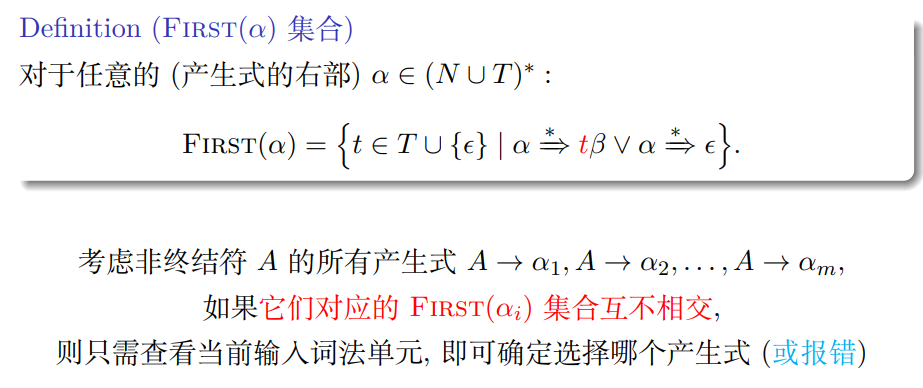#### 3.3 FOLLOW($A$)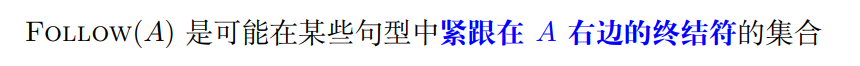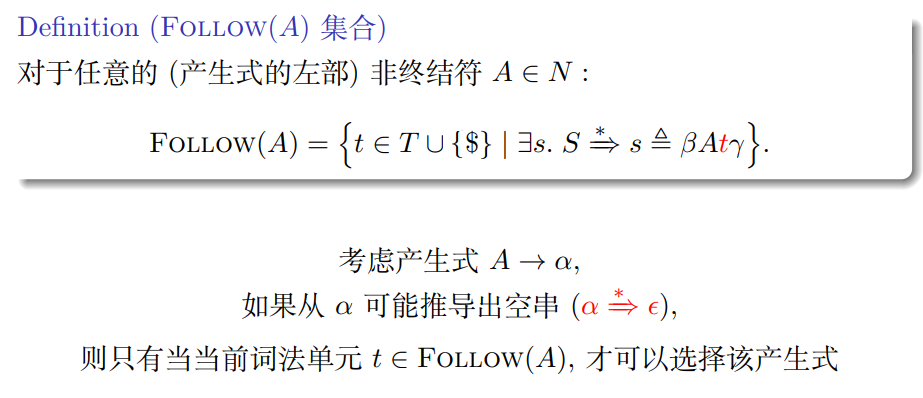#### 3.4 计算FIRST,FOLLOW

##### 3.4.1 FIRST
1.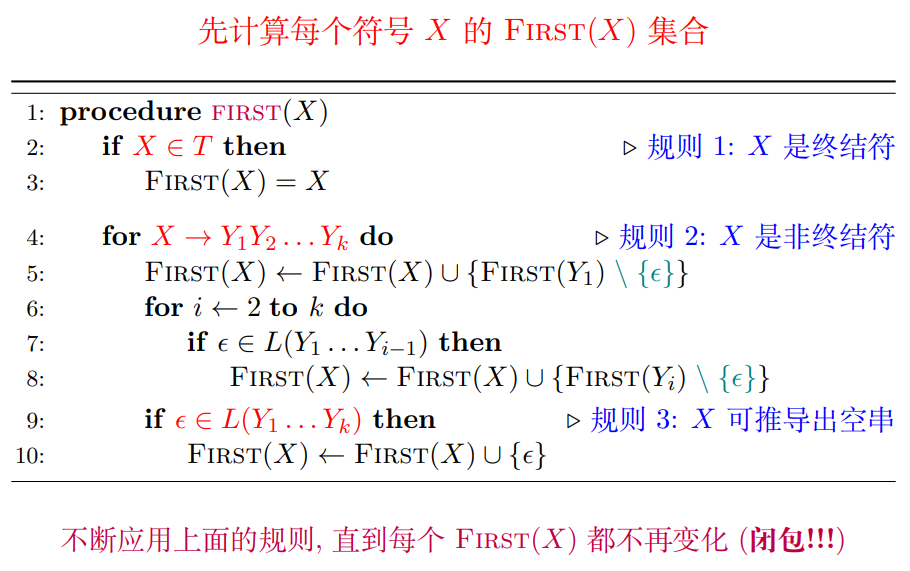- 只有前面所有非终结符都能推出 $\epsilon$, 才需要考虑下一个
2.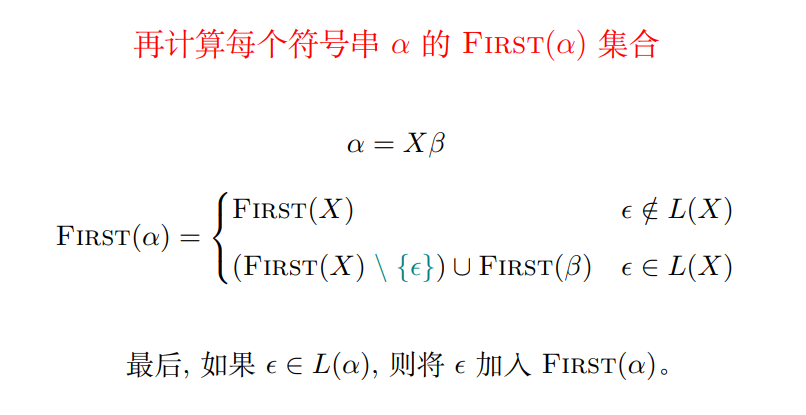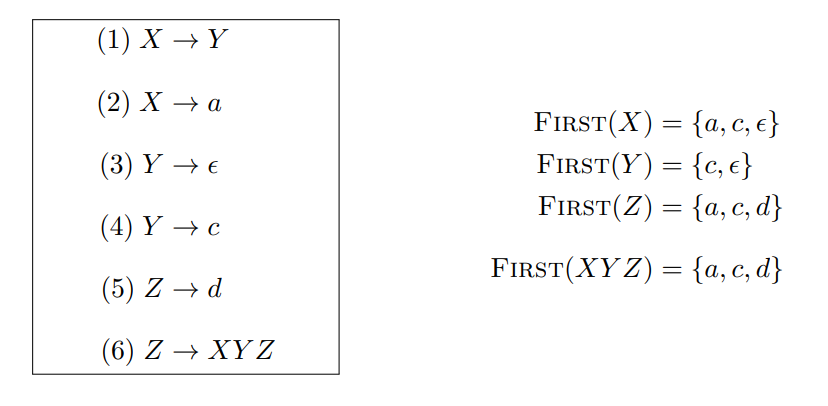##### 3.4.2 FOLLOW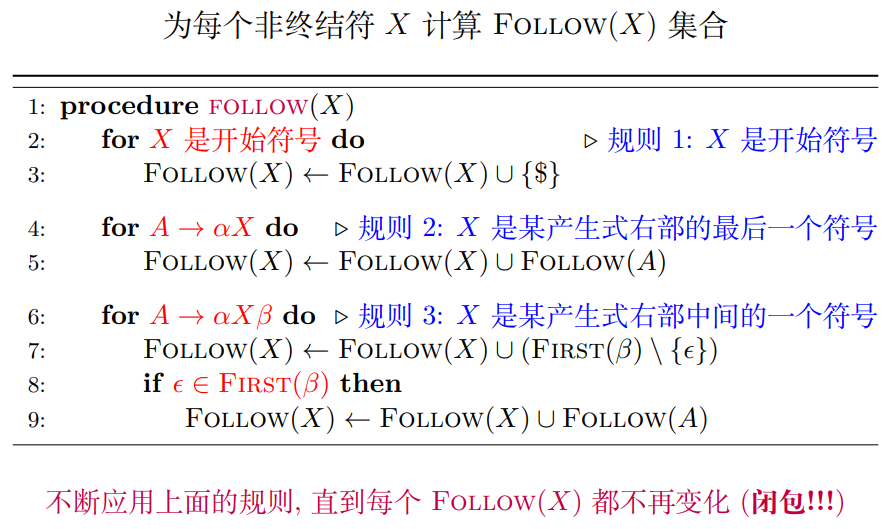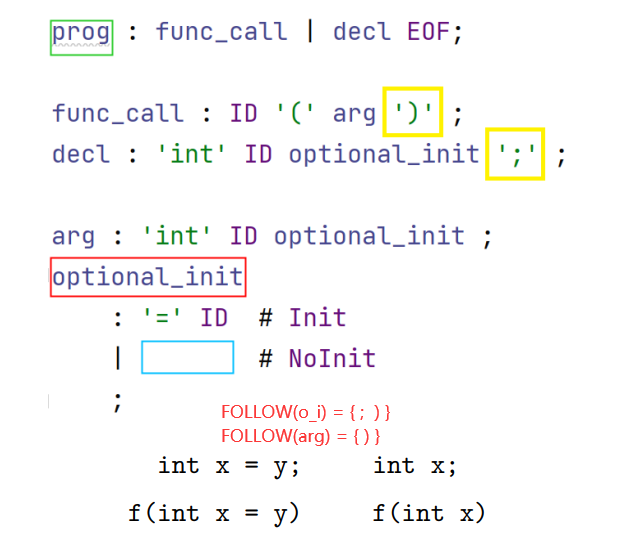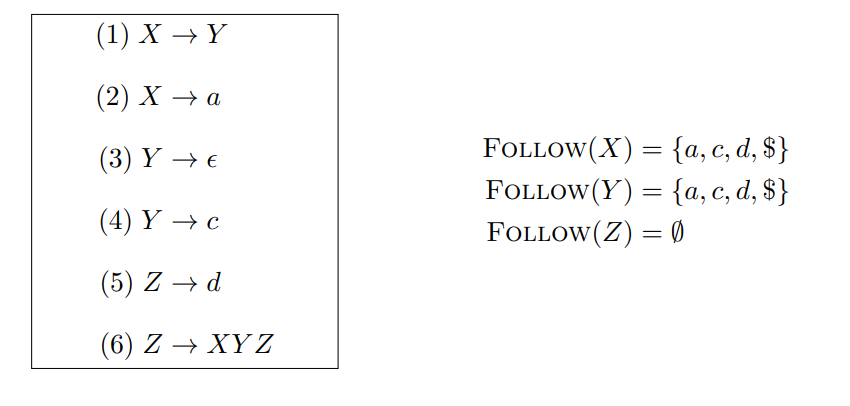#### 3.5 计算预测分析表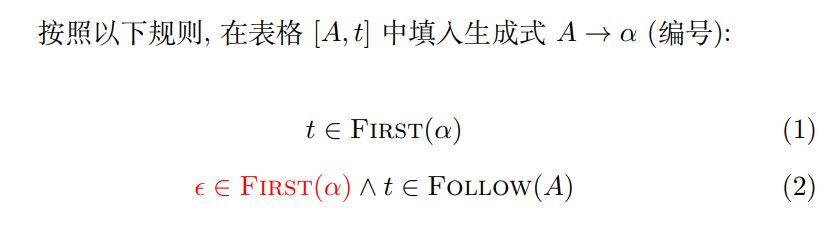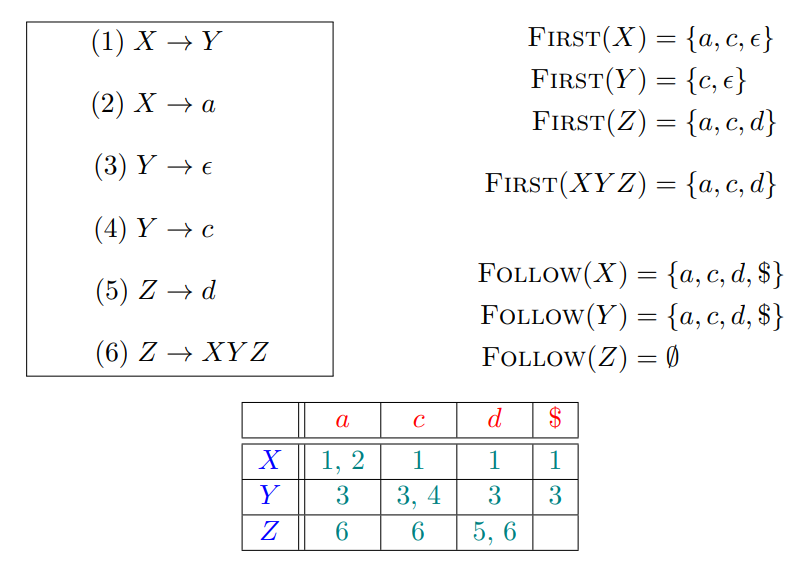### 4. 非递归的预测分析算法

S: 初始符号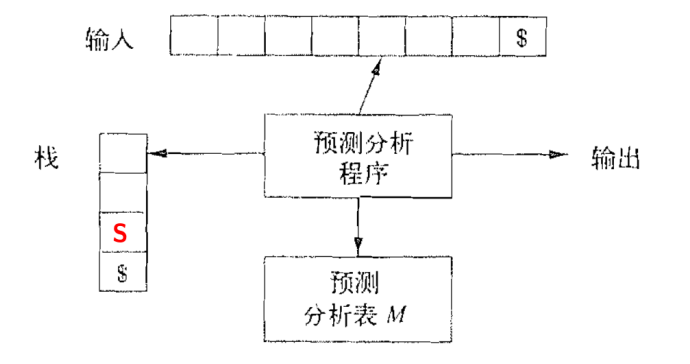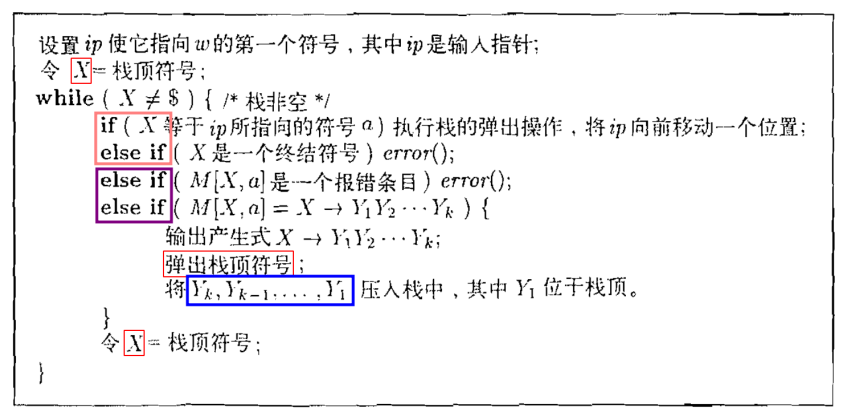### 5. 非$LL(1)$文法如何解决

• 消除左递归
• 提取左公因子

#### 5.1 提取左公因子

• antlr 不需要这么处理, 会使用更好的技术来处理

#### 5.2 消除左递归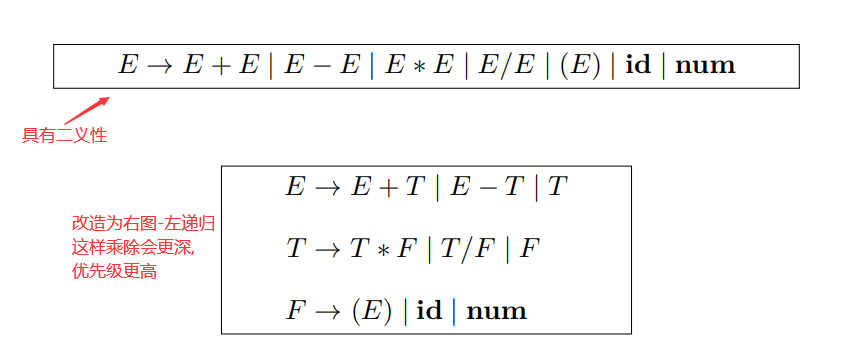• 但是左递归文法在使用$LL(1)$文法的情况下是不能运行的
• $LL(1)$文法需要为 $E$ 写一个递归函数
• $E 在 \textcolor{red}{\textbf{不消耗任何词法单元}}的情况下, 直接递归调用 E, 造成\textcolor{green}{\textbf{死循环}}$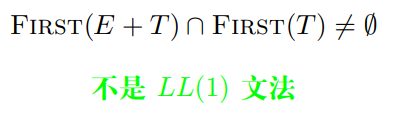##### 右递归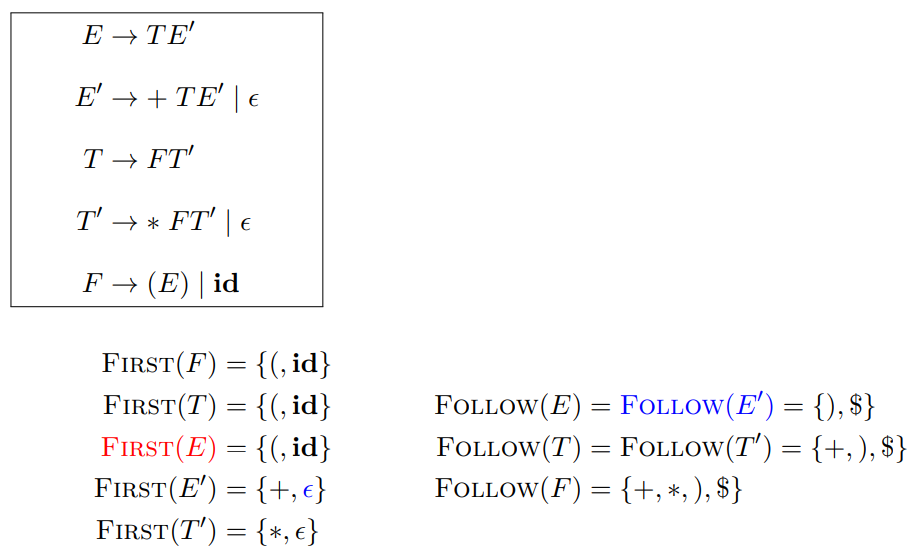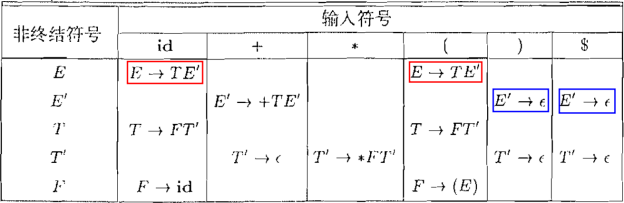## 三、Adaptive $LL(\ast)$ 语法分析算法

### 1. ANTLR 4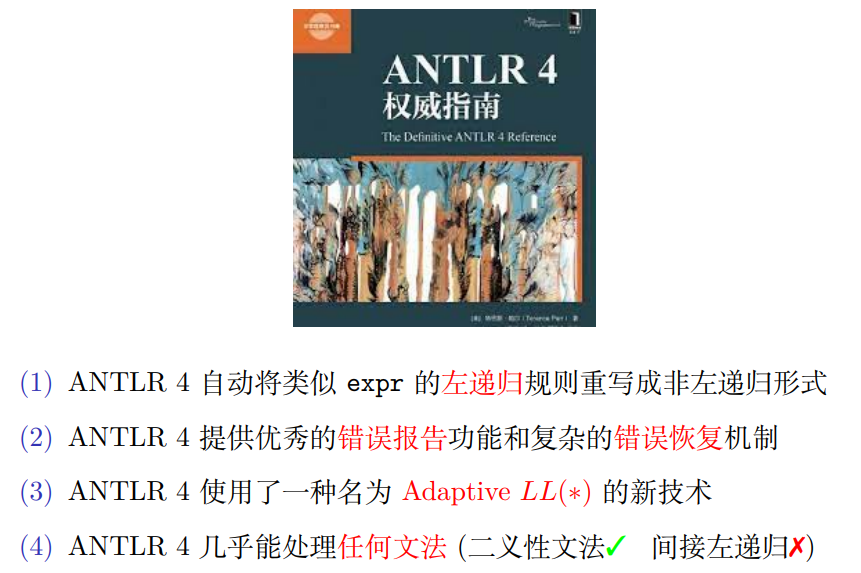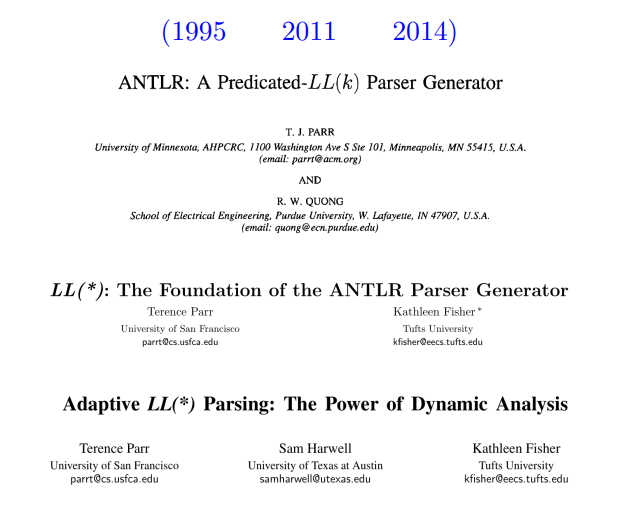courses-at-nju-by-hfwei/compilers-papers-we-love

### 2. 直接左递归与优先级

#### 2.1 引入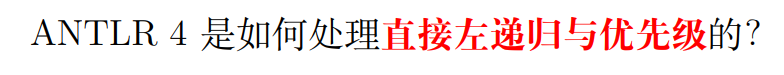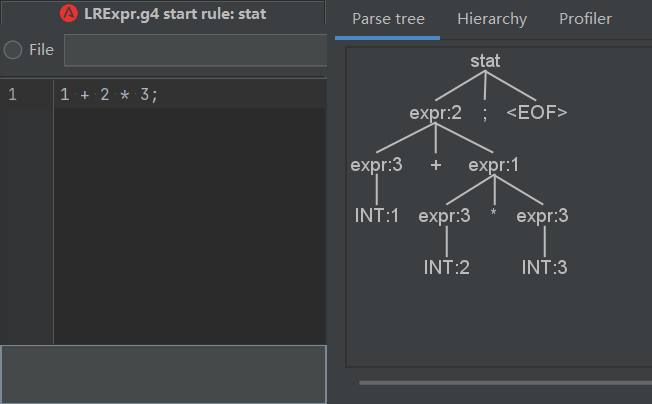• 和 + 同一层, 即上图情况, 则 * 更深, 优先级更高
• 和的父节点一层, 则 + 与 * 优先级相同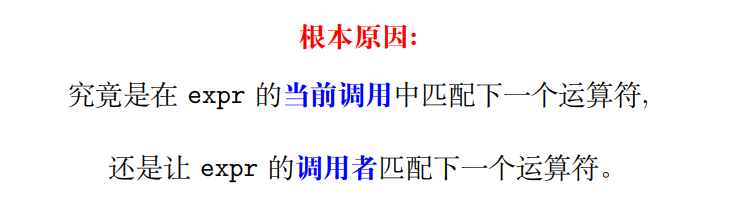#### 2.2 ANTLR 处理方法(优先级上升算法)

antlr4 LRExpr -Xlog

• 添加 -Xlog 可以查看被 antlr4 重写后的文法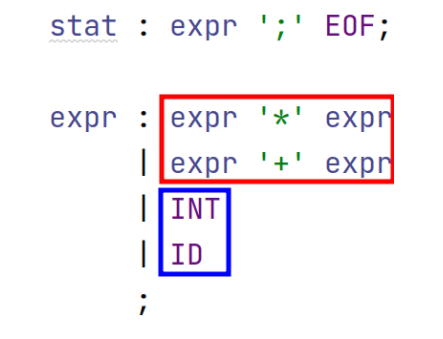$\Longrightarrow$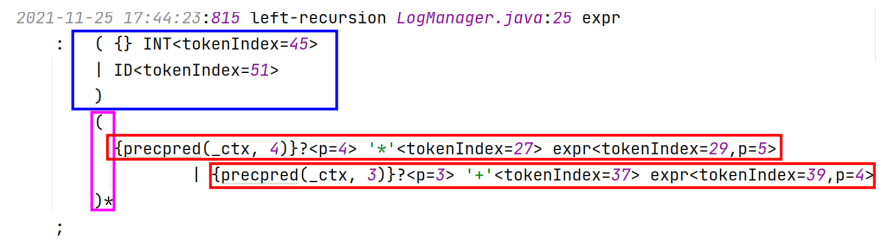$\stackrel{简化一下}{\Longrightarrow}$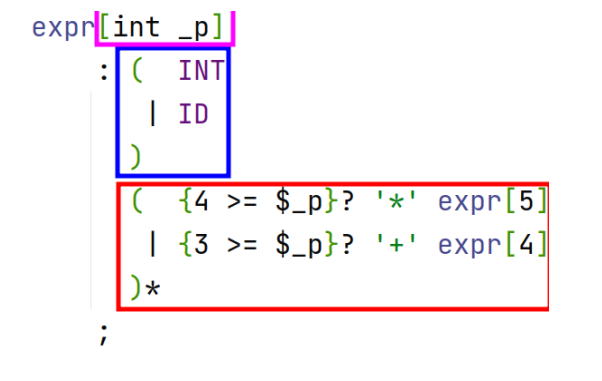• antlr4中, 优先级从上至下以此降低, 最底层优先级为 1, 每往上一层 +1
• ID-1, INT-2, (expr ‘+’ expr)-3, (expr ‘*‘ expr)-4

1 + 2 + 3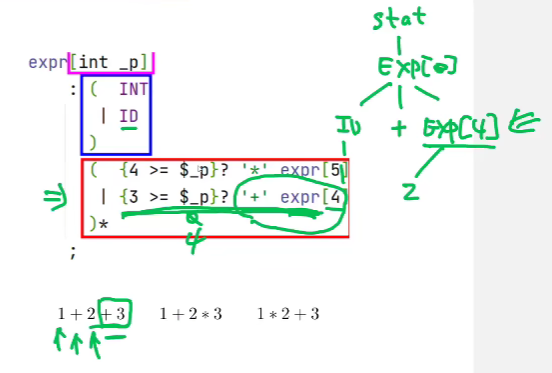• 当遇到最后的 +3 时, 因为优先级限制不能直接展开, 否则就变成右结合了

• exp 中的 4 限制了要在哪一层展开, 这一层不满足, 要返回到上一层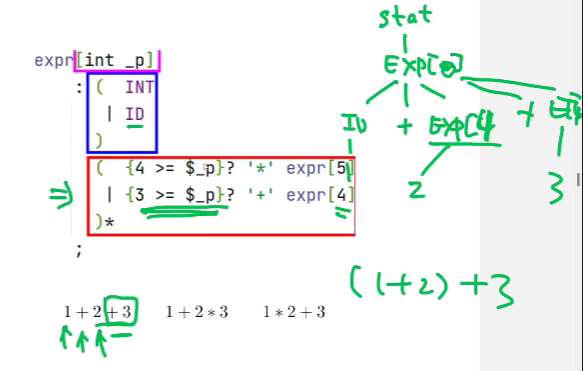• 在上一层展开 就变为 ID '+' EXP '+' EXP

1 + 2 * 3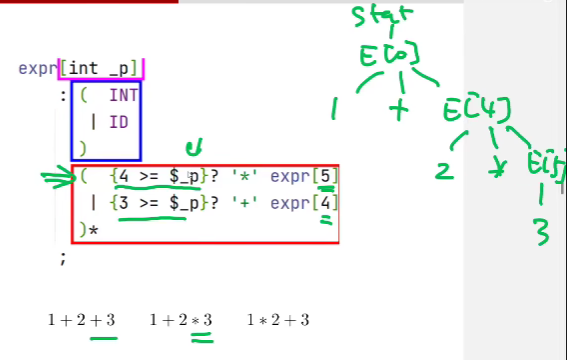1 * 2 + 3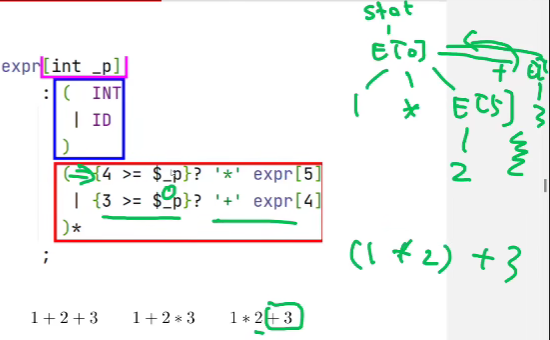#### 2.3 扩展一下语法

1.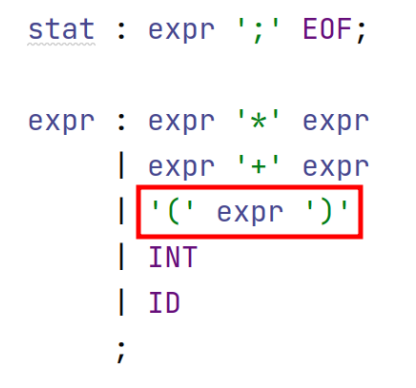- 非常简单，因为不是左递归，处理时和 INT ID 一组
2.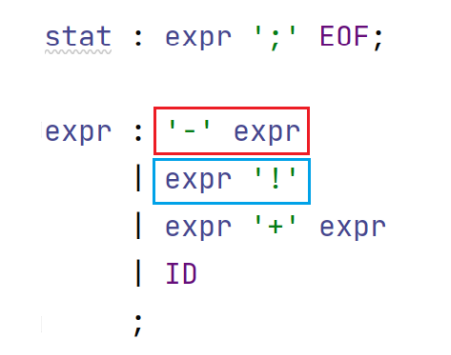• '-' expr 非左递归, 尽管优先级高, 处理时和 ID 一组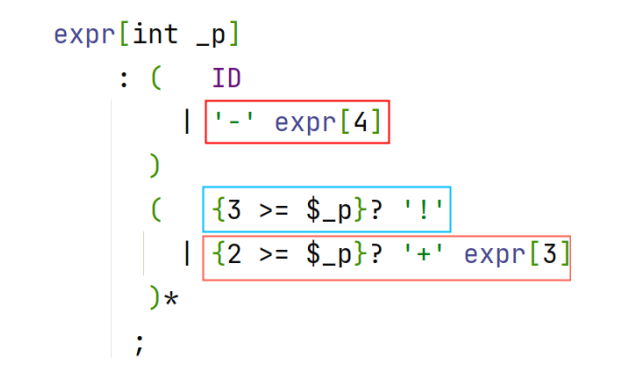-a + b! $\implies$ (-a) + (b!)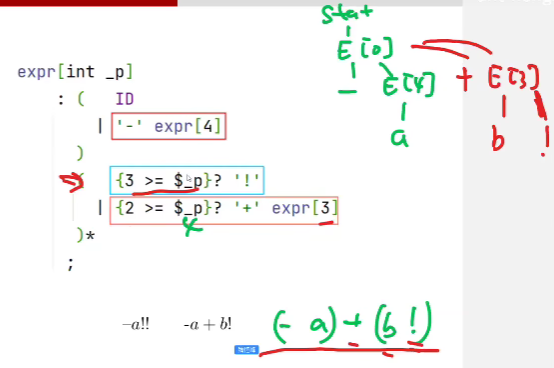-a!!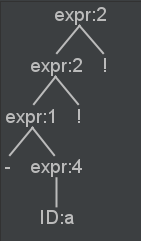1.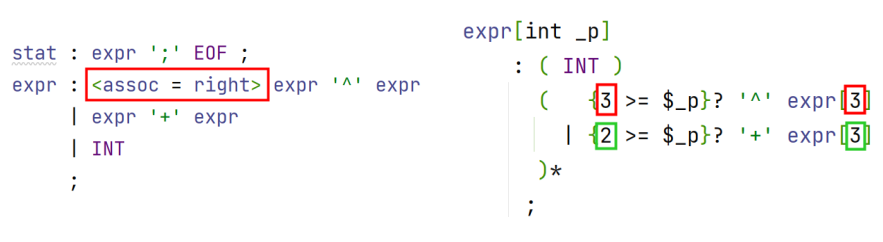- 为了实现幂运算的右结合, 推导幂运算是, expr的优先级仍然是 expr

1^2^3 + 4

#### 2.4 如何决定优先级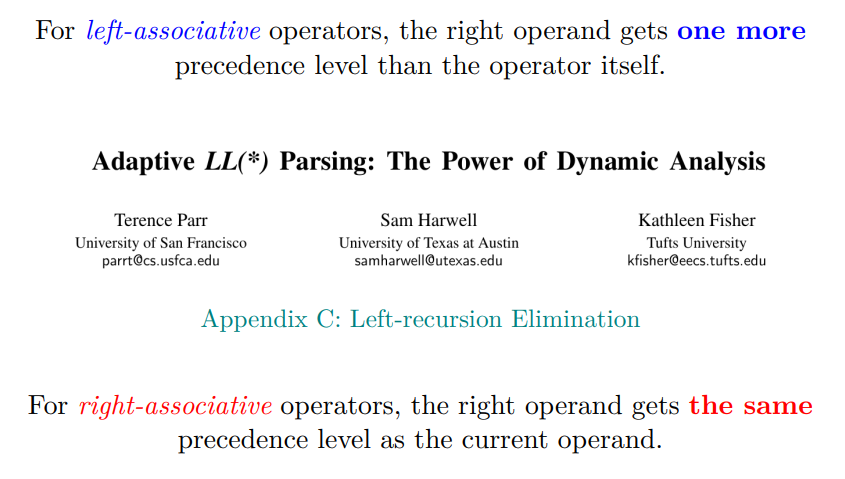### 3. 错误报告与恢复

#### 3.1 引入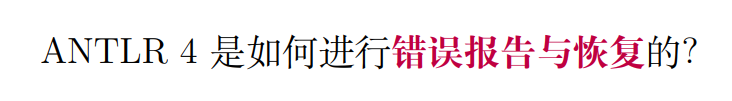• 说明不是遇到错误就停下来

#### 3.2 恐慌/应急(Panic)模式

• 假装成功、调整状态、继续进行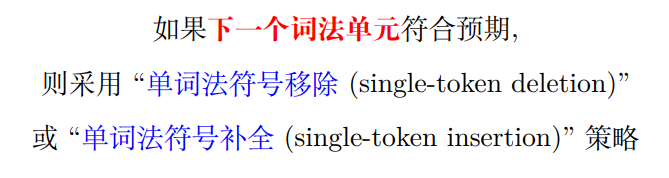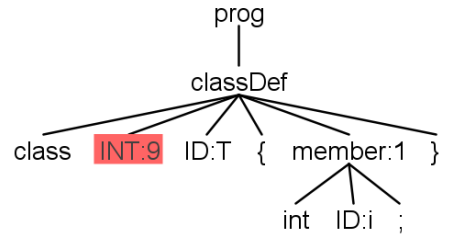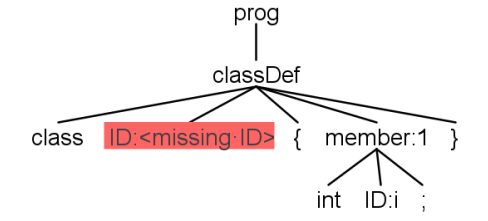• 但这种方法只能解决简单的错误, 复杂可使用 “同步-返回” 策略

#### 3.3 同步-返回 策略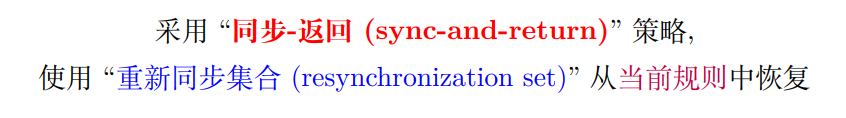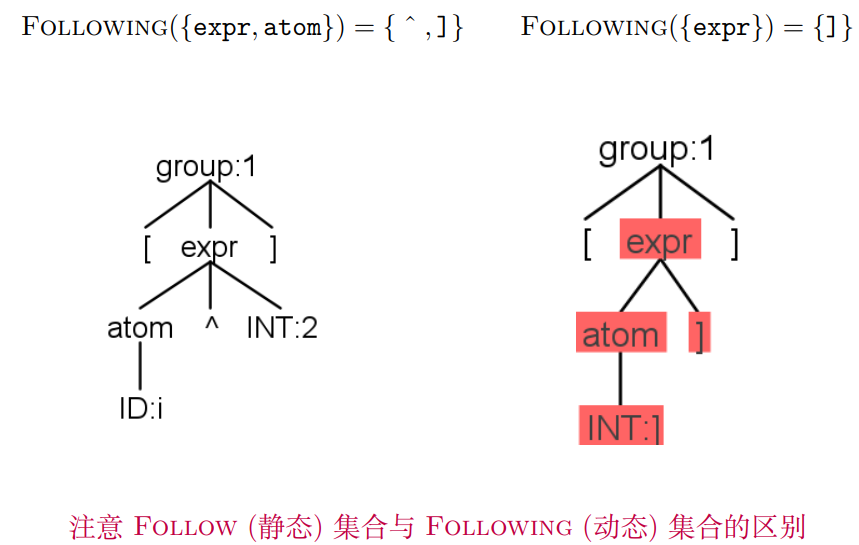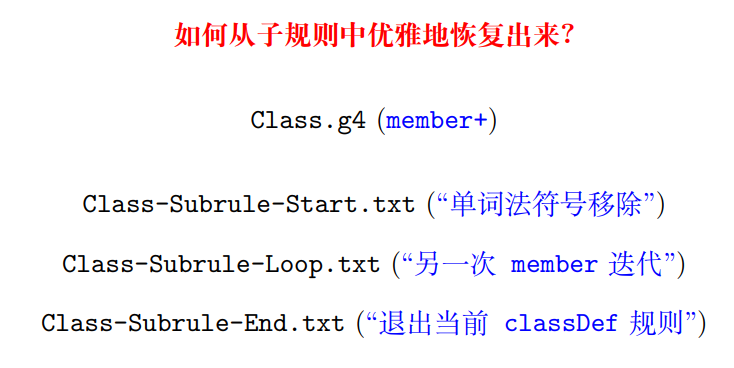### 4. Adaptive $LL(\ast)$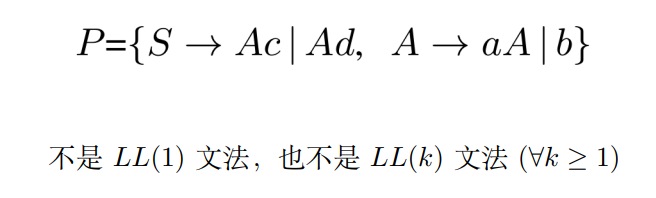$A: a^\ast b$

1. 为每个非终结符构造ATN网络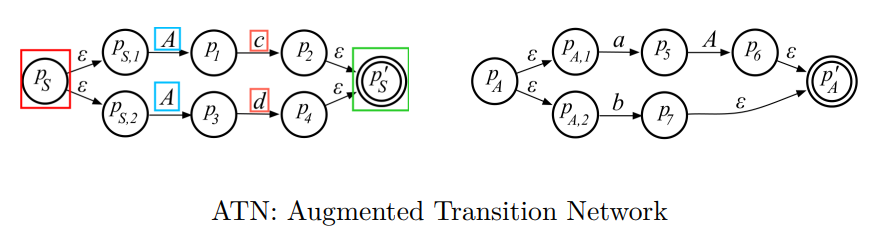1. 根据ATN网络, 构造”向前看DFA”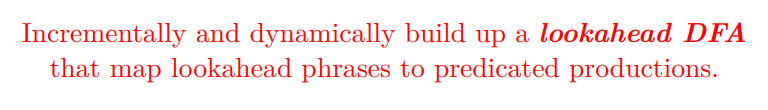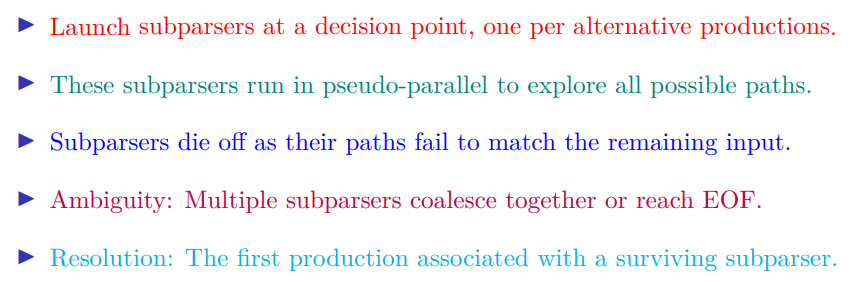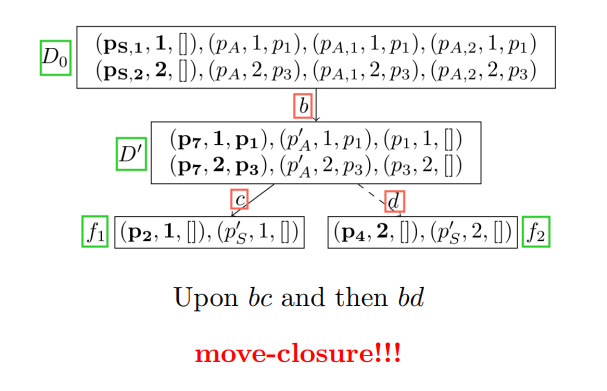$D_0$: 初始状态

$f_{1/2}$: 第1/2条备选分支

2023-08-09 14:53:19